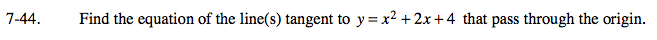### Home > CALC > Chapter 7 > Lesson 7.1.4 > Problem7-44

7-44.y = x2 + 2x + 4 has infinitely many tangent lines; two of them go through the origin.

Let the point of tangency be (a, y(a)) or (a, (a2 +2a + 4)).

Therefore, the slope of the tangent lines must be y'(a) = 2a + 2.
Of course, we do not know the value of 'a' yet.

Write the equation of the tangent line(s) using Point-Slope form. Then solve for y. We will solve for 'a' next.

Since we know that the lines pass through the origin, evaluate (x, y) for (0,0). This will give you the values of 'a'.

Use the eTool below to explore the problem.
Click the link at right for the full version of the eTool: Calc 7-44 HW eTool